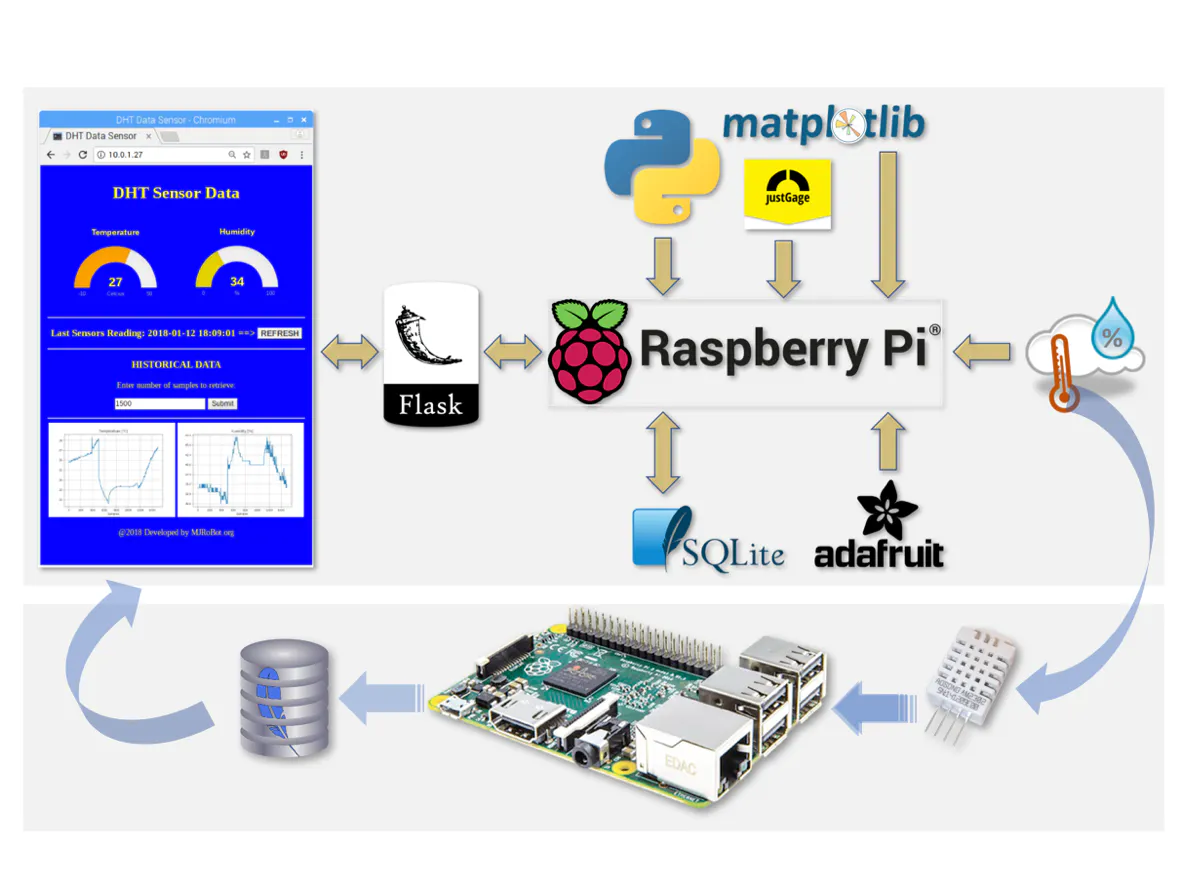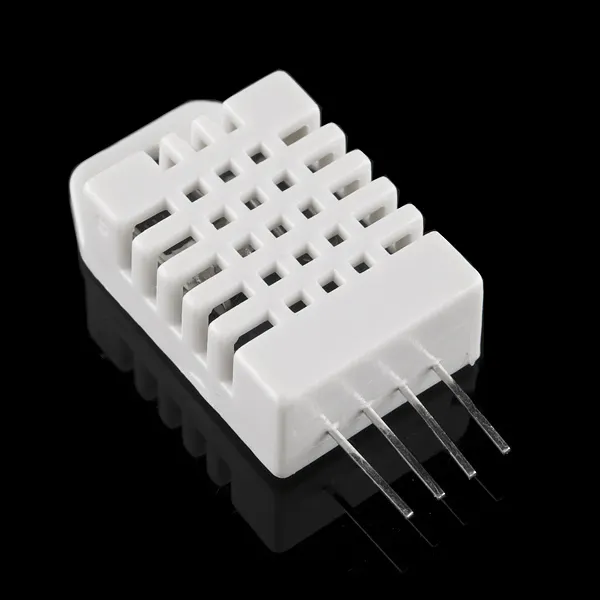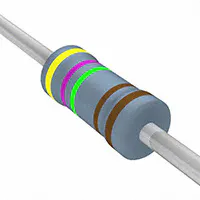# From Data to Graph: A Web Journey With Flask and SQLite

Capturing real data (RPi/DHT22), saving them in a database (SQLite), creating graphs (Matplotlib) and presenting them on a web page (Flask).

IntermediateFull instructions provided12 hours15,937## Things used in this project

### Hardware componentsRaspberry Pi 3 Model B
×1DHT22 Temperature Sensor
×1Resistor 4.75k ohm
×1

## Code

### Code snippet #9

Plain text
```import sqlite3 as lite
import sys
con = lite.connect('sensorsData.db')
with con:
cur = con.cursor()
cur.execute("DROP TABLE IF EXISTS DHT_data")
cur.execute("CREATE TABLE DHT_data(timestamp DATETIME, temp NUMERIC, hum NUMERIC)")
```

### Code snippet #14

Plain text
```import sqlite3 as lite
import sys
con = lite.connect('sensorsData.db')
with con:
cur = con.cursor()
cur.execute("INSERT INTO DHT_data VALUES(datetime('now'), 20.5, 30)")
cur.execute("INSERT INTO DHT_data VALUES(datetime('now'), 25.8, 40)")
cur.execute("INSERT INTO DHT_data VALUES(datetime('now'), 30.3, 50)")
```

### Code snippet #17

Plain text
```import sqlite3
import sys
conn=sqlite3.connect('sensorsData.db')
curs=conn.cursor()

# function to insert data on a table
curs.execute("INSERT INTO DHT_data values(datetime('now'), (?), (?))", (temp, hum))
conn.commit()

# call the function to insert data

# print database content
print ("\nEntire database contents:\n")
for row in curs.execute("SELECT * FROM DHT_data"):
print (row)

# close the database after use
conn.close()
```

### Code snippet #22

Plain text
```import Adafruit_DHT
DHTpin = 16

if humidity is not None and temperature is not None:
print('Temp={0:0.1f}*C  Humidity={1:0.1f}%'.format(temperature, humidity))
else:
print('Failed to get reading. Try again!')
```

### Code snippet #24

Plain text
```import time
import sqlite3

dbname='sensorsData.db'
sampleFreq = 2 # time in seconds

# get data from DHT sensor
def getDHTdata():
DHTpin = 16

if hum is not None and temp is not None:
hum = round(hum)
temp = round(temp, 1)
logData (temp, hum)

# log sensor data on database
def logData (temp, hum):
conn=sqlite3.connect(dbname)
curs=conn.cursor()
curs.execute("INSERT INTO DHT_data values(datetime('now'), (?), (?))", (temp, hum))
conn.commit()
conn.close()

# display database data
def displayData():
conn=sqlite3.connect(dbname)
curs=conn.cursor()
print ("\nEntire database contents:\n")
for row in curs.execute("SELECT * FROM DHT_data"):
print (row)
conn.close()

# main function
def main():
for i in range (0,3):
getDHTdata()
time.sleep(sampleFreq)
displayData()

# Execute program
main()
```

### Code snippet #26

Plain text
```import time
import sqlite3

dbname='sensorsData.db'
sampleFreq = 1*60 # time in seconds ==> Sample each 1 min

# get data from DHT sensor
def getDHTdata():
DHTpin = 16
if hum is not None and temp is not None:
hum = round(hum)
temp = round(temp, 1)
return temp, hum

# log sensor data on database
def logData (temp, hum):
conn=sqlite3.connect(dbname)
curs=conn.cursor()
curs.execute("INSERT INTO DHT_data values(datetime('now'), (?), (?))", (temp, hum))
conn.commit()
conn.close()

# main function
def main():
while True:
temp, hum = getDHTdata()
logData (temp, hum)
time.sleep(sampleFreq)

# ------------ Execute program
main()
```

### Code snippet #28

Plain text
```import sqlite3
conn=sqlite3.connect('sensorsData.db')
curs=conn.cursor()

maxTemp = 27.6

print ("\nEntire database contents:\n")
for row in curs.execute("SELECT * FROM DHT_data"):
print (row)

print ("\nDatabase entries for a specific humidity value:\n")
for row in curs.execute("SELECT * FROM DHT_data WHERE hum='29'"):
print (row)

print ("\nDatabase entries where the temperature is above 30oC:\n")
for row in curs.execute("SELECT * FROM DHT_data WHERE temp>30.0"):
print (row)

print ("\nDatabase entries where the temperature is above x:\n")
for row in curs.execute("SELECT * FROM DHT_data WHERE temp>(?)", (maxTemp,)):
print (row)
```

### Code snippet #31

Plain text
```import sqlite3
conn = sqlite3.connect('sensorsData.db')
curs=conn.cursor()

print ("\nLast Data logged on database:\n")
for row in curs.execute("SELECT * FROM DHT_data ORDER BY timestamp DESC LIMIT 1"):
print (row)
```

### Code snippet #32

Plain text
```import sqlite3
conn=sqlite3.connect('sensorsData.db')
curs=conn.cursor()

print ("\nLast raw Data logged on database:\n")
for row in curs.execute("SELECT * FROM DHT_data ORDER BY timestamp DESC LIMIT 1"):
print (str(row)+" ==> Temp = "+str(row)+"	Hum ="+str(row))
```

### Code snippet #40

Plain text
```├── Sensors_Database
├── sensorsData.db
├── logDHT.py
├── dhtWebSensor
├── templates
└── static
```

### Code snippet #41

Plain text
```from flask import Flask, render_template, request

import sqlite3

# Retrieve data from database
def getData():
conn=sqlite3.connect('../sensorsData.db')
curs=conn.cursor()

for row in curs.execute("SELECT * FROM DHT_data ORDER BY timestamp DESC LIMIT 1"):
time = str(row)
temp = row
hum = row
conn.close()
return time, temp, hum

# main route
@app.route("/")
def index():
time, temp, hum = getData()
templateData = {
'time': time,
'temp': temp,
'hum': hum
}
return render_template('index.html', **templateData)

if __name__ == "__main__":
app.run(host='0.0.0.0', port=80, debug=False)
```

### Code snippet #42

Plain text
```<!DOCTYPE html>
<title>DHT Sensor data </title>
<body>
<h1>DHT Sensor Data </h1>
<h3> TEMPERATURE   ==>  {{ tempLab  }} oC</h3>
<h3> HUMIDITY (Rel.) ==>  {{ humLab  }} %</h3>
<hr>
<h3> Last Sensors Reading: {{ time }} ==> <a href="/"class="button">REFRESH</a></h3>
<hr>
<p> @2018 Developed by MJRoBot.org</p>
</body>
</html>
```

### Code snippet #43

Plain text
```body{
background: blue;
color: yellow;
}

.button {
font: bold 15px Arial;
text-decoration: none;
background-color: #EEEEEE;
color: #333333;
border-top: 1px solid #CCCCCC;
border-right: 1px solid #333333;
border-bottom: 1px solid #333333;
border-left: 1px solid #CCCCCC;
}
```

### Code snippet #44

Plain text
```├── Sensors_Database
├── sensorsData.db
├── logDHT.py
├── dhtWebSensor
├── appDhtWebSensor.py
├── templates
│      ├── index.html
└── static
├── style.css
```

### Code snippet #47

Plain text
```<!doctype html>
<html>

<title>DHT Data Sensor</title>
<meta http-equiv="Content-Type" content="text/html; charset=UTF-8" />
<style>
body {
text-align: center;
}
#g1,
#g2 {
width: 200px;
height: 160px;
display: inline-block;
margin: 1em;
}
</style>
<body>
<h1>DHT Sensor Data </h1>
<div id="g1"></div>
<div id="g2"></div>
<hr>
<h3> Last Sensors Reading: {{ time }} ==> <a href="/"class="button">REFRESH</a></h3>
<hr>
<p> @2018 Developed by MJRoBot.org</p>

<script src="../static/raphael-2.1.4.min.js"></script>
<script src="../static/justgage.js"></script>
<script>
var g1, g2;
g1 = new JustGage({
id: "g1",
value: {{temp}},
valueFontColor: "yellow",
min: -10,
max: 50,
title: "Temperature",
label: "Celcius"
});
g2 = new JustGage({
id: "g2",
value: {{hum}},
valueFontColor: "yellow",
min: 0,
max: 100,
title: "Humidity",
label: "%"
});
});
</script>
</body>
</html>
```

### Code snippet #49

Plain text
```├── Sensors_Database
├── sensorsData.db
├── logDHT.py
├── dhtWebHist
├── appDhtWebHist.py
├── templates
│      ├── index.html
└── static
├── style.css
```

### Code snippet #50

Plain text
```from matplotlib.backends.backend_agg import FigureCanvasAgg as FigureCanvas
from matplotlib.figure import Figure
import io

import sqlite3
conn=sqlite3.connect('../sensorsData.db')
curs=conn.cursor()

global numSamples

#numSamples = maxRowsTable()
#if (numSamples > 101):
numSamples = 100

# Retrieve LAST data from database
def getLastData():
for row in curs.execute("SELECT * FROM DHT_data ORDER BY timestamp DESC LIMIT 1"):
time = str(row)
temp = row
hum = row
#conn.close()
return time, temp, hum

def getHistData (numSamples):
curs.execute("SELECT * FROM DHT_data ORDER BY timestamp DESC LIMIT "+str(numSamples))
data = curs.fetchall()
dates = []
temps = []
hums = []
for row in reversed(data):
dates.append(row)
temps.append(row)
hums.append(row)
return dates, temps, hums

def maxRowsTable():
for row in curs.execute("select COUNT(temp) from  DHT_data"):
maxNumberRows=row
return maxNumberRows

# main route
@app.route("/")
def index():
time, temp, hum = getLastData()
templateData = {
'time'	: time,
'temp'	: temp,
'hum'	: hum,
'numSamples'	: numSamples
}
return render_template('index.html', **templateData)

@app.route('/', methods=['POST'])
def my_form_post():
global numSamples
numSamples = int (request.form['numSamples'])
numMaxSamples = maxRowsTable()
if (numSamples > numMaxSamples):
numSamples = (numMaxSamples-1)
time, temp, hum = getLastData()
templateData = {
'time'	: time,
'temp'	: temp,
'hum'	: hum,
'numSamples'	: numSamples
}
return render_template('index.html', **templateData)

@app.route('/plot/temp')
def plot_temp():
times, temps, hums = getHistData(numSamples)
ys = temps
fig = Figure()
axis.set_title("Temperature [°C]")
axis.set_xlabel("Samples")
axis.grid(True)
xs = range(numSamples)
axis.plot(xs, ys)
canvas = FigureCanvas(fig)
output = io.BytesIO()
canvas.print_png(output)
response = make_response(output.getvalue())
response.mimetype = 'image/png'
return response

@app.route('/plot/hum')
def plot_hum():
times, temps, hums = getHistData(numSamples)
ys = hums
fig = Figure()
axis.set_title("Humidity [%]")
axis.set_xlabel("Samples")
axis.grid(True)
xs = range(numSamples)
axis.plot(xs, ys)
canvas = FigureCanvas(fig)
output = io.BytesIO()
canvas.print_png(output)
response = make_response(output.getvalue())
response.mimetype = 'image/png'
return response

if __name__ == "__main__":
app.run(host='0.0.0.0', port=80, debug=False)
```

### Code snippet #51

Plain text
```<!DOCTYPE html>
<title>DHT Sensor data </title>
<body>
<h1>DHT Sensor Data </h1>
<h3> TEMPERATURE   ==>  {{ temp  }} oC</h3>
<h3> HUMIDITY (Rel.) ==>  {{ hum  }} %</h3>
<hr>
<h3> Last Sensors Reading: {{ time }} ==> <a href="/"class="button">REFRESH</a></h3>
<hr>
<h3> HISTORICAL DATA </h3>
<p> Enter number of samples to retrieve:
<form method="POST">
<input name="numSamples" value= {{numSamples}}>
<input type="submit">
</form></p>
<hr>
<img src="/plot/temp" alt="Image Placeholder" width="49%">
<img src="/plot/hum" alt="Image Placeholder" width="49%">
<p> @2018 Developed by MJRoBot.org</p>
</body>
</html>
```

### Code snippet #52

Plain text
```body{
background: blue;
color: yellow;
}

.button {
font: bold 15px Arial;
text-decoration: none;
background-color: #EEEEEE;
color: #333333;
border-top: 1px solid #CCCCCC;
border-right: 1px solid #333333;
border-bottom: 1px solid #333333;
border-left: 1px solid #CCCCCC;
}

img{
display: display: inline-block
}
```